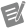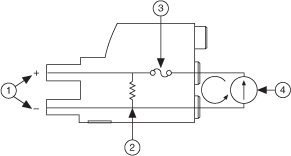Measuring Current

NI Digital Multimeters Help (NI-DMM 18.1)

Edition Date: March 2018

Part Number: 370384V-01

»View Product InfoDownload Help (Windows Only)

This section describes how to measure current on National Instruments DMMs.

Measuring Current Within the Normal Range (NI 4050 is not supported)

To configure current measurements within the normal range on the DMM, use one of the following two methods:

Method 1

1. Select the current measurement function.
2. Take a reading from the DMM immediately.

Method 2

1. Configure the DMM for a software trigger.
2. Initiate the DMM for a current measurement to cause the input relay to switch.
3. Send a software trigger to the DMM to take a measurement.

Using Current Shunt Modules

When taking current measurements outside of the specified range on the DMM, and when taking any current measurements on the NI 4050, you must use a current shunt module. NI offers the NI CSM-10A and the NI CSM-200mA current shunt modules. The NI CSM-10A has a 0.01 Ω sense resistor, and the NI CSM-200mA has a 1.0 Ω sense resistor.Note  For valid current ranges, refer to the specifications document for your device.

Each current shunt module (CSM) operates by passing the input current through a precise resistor. The following figure shows the internal circuitry of the CSM:1 Voltage Across Resistor (VOUT) 3 Fuse 2 Precision Resistor 4 Current Source (IIN)

To configure current measurements using the NI CSM-200mA or the NI CSM-10A, perform one of the following two methods:

Method 1

1. Measure the voltage drop across the resistor of the CSM.
2. Use this value to calculate the current using Ohm's Law, as follows:

IIN = (VOUT)/R

where

I is the input current

V is the voltage across the precision resistor

R is the resistance of the precision resistor

For example, assume that you are using the CSM-200mA, which has a 1 Ω precision resistor, and the measured voltage is 50 mV. Apply these values to Ohm's Law to determine the current, as follows:

50 mA = 50 mV / 1 ΩNote  You can use the NI CSM-10A current shunt with any NI DMM for this method. You can also use the NI CSM-200mA current shunt with the NI 4050.

Method 2 (NI 4050 only)

For LabVIEW:

1. Call niDMM Config Measurement.
2. Specify the shunt value in the Shunt Value property node.
3. Call niDMM Read to obtain the value of the measurement.

For C/C++/CVI/Visual Basic:

1. Call niDMM_ConfigureMeasurementDigits.
2. Specify the shunt value in the NIDMM_ATTR_SHUNT_VALUE attribute.